• 利用分水岭算法进行图像分割matlab实现 1’原理 分水岭算法图像看成“地形图”，灰度值大的对应山峰，灰度值小的对应集水盆，集水盆边界即为分水岭。 在每一个局部极小值表面，刺穿一个小孔，然后把整个模型慢慢浸...
利用分水岭算法进行图像分割matlab实现
1’原理
分水岭算法将图像看成“地形图”，灰度值大的对应山峰，灰度值小的对应集水盆，集水盆边界即为分水岭。
在每一个局部极小值表面，刺穿一个小孔，然后把整个模型慢慢浸入水中，随着浸入的加深，每一个局部极小值的影响域慢慢向外扩展，在两个集水盆汇合处构筑大坝，即形成分水岭。
2’步骤
1’’读取图像转化为灰度图
2’’求图像梯度，对梯度应用分水岭算法（容易过度分割，效果不佳）
或者对图像前景和背景进行标记
3’’对图像计算分水岭
3’实现
%%
clear;clc;close all;
img_gray=imread('gantrycrane.png');%读取图片
img_gray=rgb2gray(img_gray);
%% 求梯度,对梯度图像求分水岭
% img_gray_=double(img_gray);
% [GX,GY]=mygradient(img_gray_);
% img_grad=sqrt(GX.*GX+GY.*GY);
% img_blur=myblur(img_grad);
% img_blur=uint8(img_blur);
% water_line__=watershed(img_blur);           %
% water_line_=(water_line__==0)
% imshow(water_line_,[])
%% 腐蚀膨胀，基于标记的分水岭
se = strel('disk',2);
Ie = imerode(img_gray, se);
Iobr = imreconstruct(Ie, img_gray);
Iobrd = imdilate(Iobr, se);
Iobrcbr = imreconstruct(imcomplement(Iobrd), imcomplement(Iobr));
Iobrcbr = imcomplement(Iobrcbr);
bw = imbinarize(Iobrcbr, graythresh(Iobrcbr));
D = bwdist(bw);
D=uint8(D);
%% 获取分水岭

water_line__=watershed(D);
water_line_=(water_line__==0)
imshow(water_line_,[])




展开全文计算机视觉 后端
• 分水岭算法是一种图像区域分割法，分割的过程中将图片转化为灰度图，然后我会将灰度值看作是海拔，然后向较低点注水，这种基于地形学的解释，我们着重考虑三种点： 极小值点，该点对应一个盆地的最低点，当我们在...
一、简介

分水岭算法是一种图像区域分割法，分割的过程中将图片转化为灰度图，然后我会将灰度值看作是海拔，然后向较低点注水，这种基于地形学的解释，我们着重考虑三种点：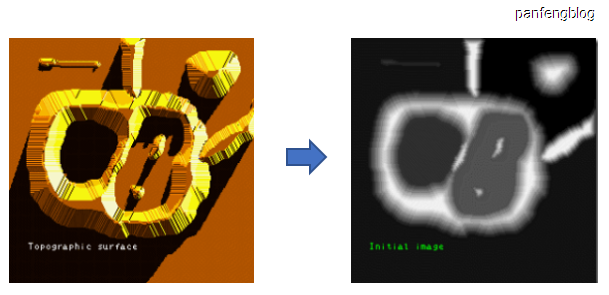极小值点，该点对应一个盆地的最低点，当我们在盆地里滴一滴水的时候，由于重力作用，水最终会汇聚到该点。注意：可能存在一个最小值面，该平面内的都是极小值点。
盆地的其它位置点，该位置滴的水滴会汇聚到局部最小点。
盆地的边缘点，是该盆地和其它盆地交接点，在该点滴一滴水，会等概率的流向任何一个盆地。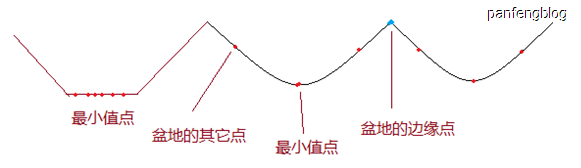明白上述三种点之后，我们开始往盆地的极小值点注水，然后随着注水的深入，每一个极小值点慢慢的向外扩展，然后知道两个盆地的水汇合，汇合处就是我们需要的分水岭。

从下图可以直观理解一下，首先这三块区域都含有极小值点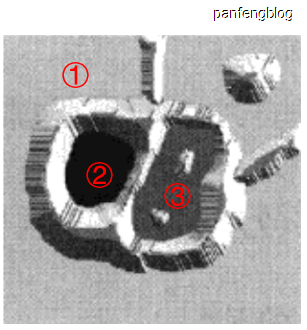然后逐渐填充就能获得分水岭（即分界线）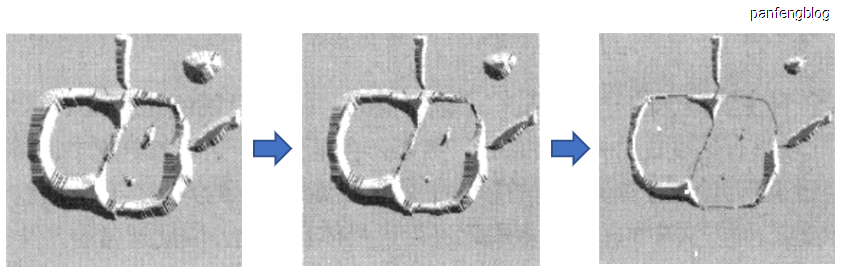得到分界线就能完成图像分割：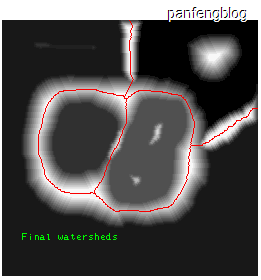二、源代码

clear, close all;
clc;
%1.读取图像并求取图像的边界。

rgb = imread('tree.jpeg');%读取原图像
I = rgb2gray(rgb);%转化为灰度图像
figure; subplot(121)%显示灰度图像
imshow(I)
text(732,501,'Image courtesy of Corel','FontSize',7,'HorizontalAlignment','right')
hy = fspecial('sobel');%sobel算子,应用sobel算子锐化图像
hx = hy';
Iy = imfilter(double(I), hy, 'replicate');%滤波求y方向边缘
Ix = imfilter(double(I), hx, 'replicate');%滤波求x方向边缘
gradmag = sqrt(Ix.^2 + Iy.^2);%求摸
subplot(122); imshow(gradmag,[]), %显示梯度
title('Gradient magnitude (gradmag)')

%2. 直接使用梯度模值进行分水岭算法：（往往会存在过的分割的情况，效果不好）

L = watershed(gradmag);%直接应用分水岭算法
Lrgb = label2rgb(L);%转化为彩色图像
figure; imshow(Lrgb), %显示分割后的图像
title('Watershed transform of gradient magnitude (Lrgb)')%过分割现象

%3.分别对前景和背景进行标记：本例中使用形态学重建技术对前景对象进行标记，首先使用开操作，开操作之后可以去掉一些很小的目标。
%开和闭这两种运算可以除去比结构元素小的特定图像细节，同时保证不产生全局几何失真。
%开运算可以把比结构元素小的突刺滤掉，切断细长搭接而起到分离作用；
%闭运算可以把比结构元素小的缺口或孔填充上，搭接短的间断而起到连接作用。
se = strel('disk', 4);%圆形结构元素,STREL('disk',R,N),R is the specified radius, When N is greater than 0, the disk-shaped structuring
%element is approximated by a sequence of N
Io = imopen(I, se);%形态学开操作
figure; subplot(121)
imshow(Io), %显示执行开操作后的图像
title('Opening (Io)')
Ie = imerode(I, se);%对图像进行腐蚀，基本参数：待处理的输入图像以及结构元素对象
Iobr = imreconstruct(Ie, I);%形态学重建
subplot(122); imshow(Iobr), %显示重建后的图像
title('Opening-by-reconstruction (Iobr)')
Ioc = imclose(Io, se);%形态学关操作，首先膨胀,然后腐蚀,两个操作使用同样的结构元素
figure; subplot(121)
imshow(Ioc), %显示关操作后的图像
title('Opening-closing (Ioc)')
Iobrd = imdilate(Iobr, se);%对图像进行膨胀，基本参数：待处理的输入图像和结构元素对象。
Iobrcbr = imreconstruct(imcomplement(Iobrd), ...
imcomplement(Iobr));%形态学重建
Iobrcbr = imcomplement(Iobrcbr);%图像求反
subplot(122); imshow(Iobrcbr), %显示重建求反后的图像,figure4
title('Opening-closing by reconstruction (Iobrcbr)')
%As you can see by comparing Iobrcbr with Ioc,
%reconstruction-based opening and closing are more
%effective than standard opening and closing at removing
%small blemishes without affecting the overall
%shapes of the objects. Calculate the regional maxima
%of Iobrcbr to obtain good foreground markers.
fgm = imregionalmax(Iobrcbr);%局部极大值
figure; imshow(fgm), %显示重建后局部极大值图像,figure5
title('Regional maxima of opening-closing by reconstruction (fgm)')
I2 = I; %前景标记图与原图叠加
I2(fgm) = 255;%局部极大值处像素值设为255
figure; imshow(I2), %在原图上显示极大值区域,figure6
title('Regional maxima superimposed on original image (I2)')
se2 = strel(ones(3,3));%结构元素
fgm2 = imclose(fgm, se2);%关操作
fgm3 = imerode(fgm2, se2);%腐蚀
fgm4 = bwareaopen(fgm3, 20);%开操作
I3 = I;
I3(fgm4) = 255;%前景处设置为255
figure; subplot(121)
imshow(I3)%显示修改后的极大值区域,figure7
title('Modified regional maxima')
bw = im2bw(Iobrcbr, graythresh(Iobrcbr));%转化为二值图像
subplot(122); imshow(bw), %显示二值图像,figure7
title('Thresholded opening-closing by reconstruction')

%4. 进行分水岭变换并显示：

D = bwdist(bw);%计算距离
DL = watershed(D);%分水岭变换
bgm = DL == 0;%求取分割边界

三、运行结果四、备注

完整代码或者仿真咨询添加QQ1575304183

展开全文• matlab实现分水岭算法处理图像分割
分享一下我老师大神的人工智能教程！零基础，通俗易懂！http://blog.csdn.net/jiangjunshow也欢迎大家转载本篇文章。分享知识，造福人民，实现我们中华民族伟大复兴！                此程序为优化后的分水岭算法，避免了图像过分割I= imread('D:\Images\pic_loc\1870405130305041503.jpg');    imshow(I);h=fspecial('sobel'); %h = fspecial(type) creates a two-dimensional filter h of the specified type. fspecial returns h as                     %a correlation kernel, which is the appropriate form to use with imfilter. type is a string having one of these values. fd=double(I);%double使数据变成双精度g=sqrt(imfilter(fd,h,'replicate').^2+imfilter(fd,h','replicate').^2);figure;imshow(g);g2=imclose(imopen(g,ones(3,3)),ones(3,3));figure;imshow(g2);im=imextendedmin(g2,10);   %Lim=watershed(bwdist(im)); %watershed分水岭算法 Lim的值greater than or equal to 0，等于0是分水岭脊像素em=Lim==0;g3=imimposemin(g2,im|em);g4=watershed(g3);figure;imshow(g4);g5=I;g5(g4==0)=255;figure;imshow(g5);实验结果：给我老师的人工智能教程打call！http://blog.csdn.net/jiangjunshow展开全文• 分水岭算法是一种图像区域分割法，分割的过程中将图片转化为灰度图，然后我会将灰度值看作是海拔，然后向较低点注水，这种基于地形学的解释，我们着重考虑三种点： 极小值点，该点对应一个盆地的最低点，当我们在...
一、简介
分水岭算法是一种图像区域分割法，分割的过程中将图片转化为灰度图，然后我会将灰度值看作是海拔，然后向较低点注水，这种基于地形学的解释，我们着重考虑三种点：极小值点，该点对应一个盆地的最低点，当我们在盆地里滴一滴水的时候，由于重力作用，水最终会汇聚到该点。注意：可能存在一个最小值面，该平面内的都是极小值点。
盆地的其它位置点，该位置滴的水滴会汇聚到局部最小点。
盆地的边缘点，是该盆地和其它盆地交接点，在该点滴一滴水，会等概率的流向任何一个盆地。明白上述三种点之后，我们开始往盆地的极小值点注水，然后随着注水的深入，每一个极小值点慢慢的向外扩展，然后知道两个盆地的水汇合，汇合处就是我们需要的分水岭。
从下图可以直观理解一下，首先这三块区域都含有极小值点然后逐渐填充就能获得分水岭（即分界线）得到分界线就能完成图像分割：二、源代码
clear, close all;
clc;
%1.读取图像并求取图像的边界。

rgb = imread('tree.jpeg');%读取原图像
I = rgb2gray(rgb);%转化为灰度图像
figure; subplot(121)%显示灰度图像
imshow(I)
text(732,501,'Image courtesy of Corel','FontSize',7,'HorizontalAlignment','right')
hy = fspecial('sobel');%sobel算子,应用sobel算子锐化图像
hx = hy';
Iy = imfilter(double(I), hy, 'replicate');%滤波求y方向边缘
Ix = imfilter(double(I), hx, 'replicate');%滤波求x方向边缘
gradmag = sqrt(Ix.^2 + Iy.^2);%求摸
subplot(122); imshow(gradmag,[]), %显示梯度
title('Gradient magnitude (gradmag)')

%2. 直接使用梯度模值进行分水岭算法：（往往会存在过的分割的情况，效果不好）

L = watershed(gradmag);%直接应用分水岭算法
Lrgb = label2rgb(L);%转化为彩色图像
figure; imshow(Lrgb), %显示分割后的图像
title('Watershed transform of gradient magnitude (Lrgb)')%过分割现象

%3.分别对前景和背景进行标记：本例中使用形态学重建技术对前景对象进行标记，首先使用开操作，开操作之后可以去掉一些很小的目标。
%开和闭这两种运算可以除去比结构元素小的特定图像细节，同时保证不产生全局几何失真。
%开运算可以把比结构元素小的突刺滤掉，切断细长搭接而起到分离作用；
%闭运算可以把比结构元素小的缺口或孔填充上，搭接短的间断而起到连接作用。
se = strel('disk', 4);%圆形结构元素,STREL('disk',R,N),R is the specified radius, When N is greater than 0, the disk-shaped structuring
%element is approximated by a sequence of N
Io = imopen(I, se);%形态学开操作
figure; subplot(121)
imshow(Io), %显示执行开操作后的图像
title('Opening (Io)')
Ie = imerode(I, se);%对图像进行腐蚀，基本参数：待处理的输入图像以及结构元素对象
Iobr = imreconstruct(Ie, I);%形态学重建
subplot(122); imshow(Iobr), %显示重建后的图像
title('Opening-by-reconstruction (Iobr)')
Ioc = imclose(Io, se);%形态学关操作，首先膨胀,然后腐蚀,两个操作使用同样的结构元素
figure; subplot(121)
imshow(Ioc), %显示关操作后的图像
title('Opening-closing (Ioc)')
Iobrd = imdilate(Iobr, se);%对图像进行膨胀，基本参数：待处理的输入图像和结构元素对象。
Iobrcbr = imreconstruct(imcomplement(Iobrd), ...
imcomplement(Iobr));%形态学重建
Iobrcbr = imcomplement(Iobrcbr);%图像求反
subplot(122); imshow(Iobrcbr), %显示重建求反后的图像,figure4
title('Opening-closing by reconstruction (Iobrcbr)')
%As you can see by comparing Iobrcbr with Ioc,
%reconstruction-based opening and closing are more
%effective than standard opening and closing at removing
%small blemishes without affecting the overall
%shapes of the objects. Calculate the regional maxima
%of Iobrcbr to obtain good foreground markers.
fgm = imregionalmax(Iobrcbr);%局部极大值
figure; imshow(fgm), %显示重建后局部极大值图像,figure5
title('Regional maxima of opening-closing by reconstruction (fgm)')
I2 = I; %前景标记图与原图叠加
I2(fgm) = 255;%局部极大值处像素值设为255
figure; imshow(I2), %在原图上显示极大值区域,figure6
title('Regional maxima superimposed on original image (I2)')
se2 = strel(ones(3,3));%结构元素
fgm2 = imclose(fgm, se2);%关操作
fgm3 = imerode(fgm2, se2);%腐蚀
fgm4 = bwareaopen(fgm3, 20);%开操作
I3 = I;
I3(fgm4) = 255;%前景处设置为255
figure; subplot(121)
imshow(I3)%显示修改后的极大值区域,figure7
title('Modified regional maxima')
bw = im2bw(Iobrcbr, graythresh(Iobrcbr));%转化为二值图像
subplot(122); imshow(bw), %显示二值图像,figure7
title('Thresholded opening-closing by reconstruction')

%4. 进行分水岭变换并显示：

D = bwdist(bw);%计算距离
DL = watershed(D);%分水岭变换
bgm = DL == 0;%求取分割边界

三、运行结果四、备注
版本：2014a


展开全文• matlab基于分水岭算法处理图像分割的源程序，其中有不同的方法，是我收集过来效果比较好的，有利于大家的学习交流
• 代码实现： f=imread('C:\Users\lenovo\... % 读入图像 figure,imshow(f); % 显示原始图像 title('原始图像'); bw=imbinarize(f,graythresh(f)); % 转换为黑白二值图像 figure; imshow(bw); title('二值图像')...
• 分水岭算法是一种图像区域分割法，分割的过程中将图片转化为灰度图，然后我会将灰度值看作是海拔，然后向较低点注水，这种基于地形学的解释，我们着重考虑三种点： 极小值点，该点对应一个盆地的最低点，当我们在...
• 数字图像处理 图像分割分水岭算法 源代码 matlab
• 此程序为优化后的分水岭算法，避免了图像分割 I= imread('D:\Images\pic_loc\1870405130305041503.jpg'); imshow(I);h=fspecial('sobel'); %h = fspecial(type) creates a two-dimensional filter h of ...
• 应用标记符控制分水岭分割 有效解决了分水岭算法图像分割中的过分割问题
• 首先对图像进行开闭腐蚀等处理,并进行形态学重建,最后利用分水岭算法对苹果进行了有效的分割
• 分水岭算法是现在医学图像分割的经典算法，具有非常高的参考价值
• 分水岭算法是一种图像区域分割法，分割的过程中将图片转化为灰度图，然后我会将灰度值看作是海拔，然后向较低点注水，这种基于地形学的解释，我们着重考虑三种点： 极小值点，该点对应一个盆地的最低点，当我们在...
• 此处为用分水岭算法实现MATLAB图像分割，在实际运用中有较强的实用价值
• matlab实现图像分水岭分割算法并且有改进的算法实现，两者相互比较效果，并且文档最后直接复制了全部的实现代码
• 利用了分水岭算法的原理及特点, 针对算法所产生的图像分割问题,采用形态学处理函数,以典型的 pears图像为例,用MATLAB 7. 0图像处理软件,进行了仿真研究。结果表明,利用分水岭算法和形态学处理函数,使得图像 中相连...
• 算法基于 Robert J 等人的“Combined Morphological........Segmentation”。 阿尔。 IEEE 翻译在 Image Proc.，第 14 卷，第 1 期。 2005 年 1 月。 dtwct的matlab函数可以从...
• 分水岭算法是一种图像区域分割法，分割的过程中将图片转化为灰度图，然后我会将灰度值看作是海拔，然后向较低点注水，这种基于地形学的解释。大佬链接：https://blog.csdn.net/TIQCmatlab/article/details/114077957...opencv 计算机视觉 边缘检测
• 《CV：AMA》一书第九章强调了matlab分水岭算法图像分割 更新： dalao说一般在进行分水岭前都要灰度化、滤波、边缘检测、轮廓查找然后应用库里的函数，解决过度分割的话要有预先标记的先验知识，一般是mark几...
• matlab编写的分水岭算法实验代码，用于图像分割处理，针对连接在一起的物体图像，进行分割效果较好
• 分水岭算法是一种图像区域分割法，分割的过程中将图片转化为灰度图，然后我会将灰度值看作是海拔，然后向较低点注水，这种基于地形学的解释，我们着重考虑三种点： 极小值点，该点对应一个盆地的最低点，当我们在...# 分水岭算法分割图像matlabmatlab 订阅